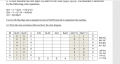# Use two JK flip-flops and a minimal two-level NAND network to implement the machine. (i). Write t

#### audreyeckman

Joined Jan 7, 2016
8
4. A state machine has one input x(t) and two-bit state (Q1(t), Q0(t)). The machine is described by the following state equations.

Q1(t + 1) = Q0(t) + x′(t) Q′1(t)

Q0(t + 1) = Q′1 (t) + x(t)Q0(t)

y(t) = Q1(t)Q0(t)

Use two JK flip-flops and a minimal two-level NAND network to implement the machine.

(i). Write the state excitation table and draw the state diagram.The above table is what I have so far, Can someone please help guide me on the JK flip flop part of this excitation table?

#### WBahn

Joined Mar 31, 2012
27,866
Well, if Q1 is presently a 0 and you need it to become a 1, what do J1 and K1 need to be in order to make that happen?

#### RBR1317

Joined Nov 13, 2010
706
While never having used excitation tables, I did learn a technique for designing sequential counters with JK flip-flops called transition mapping. However, not being certain it would be useful for designing a state machine with autonomous controls, I tried to apply it to this problem.

Each sequential variable is analyzed for each state to determine its transition to the next state. Transitions are labeled 0,1,α,β according to the following rules: 0→0=0, 1→1=1, 0→1=α, 1→0=β. States which are absent from the state table can be marked as "Don't Care" conditions, but that is not applicable to this particular problem. Then the transitions for each state variable are plotted on a Karnaugh map, and minimized for the JK logic functions. For 'J' the α are required; β,1,DC are optional. For 'K' the β are required; α,0,DC are optional.

Once the JK logic is determined, one can use the Boolean logic functions and the JK flip-flop characteristic equation in a spreadsheet or logic simulator to check whether the final result will recreate the original state table.

#### Attachments

• 42.4 KB Views: 1